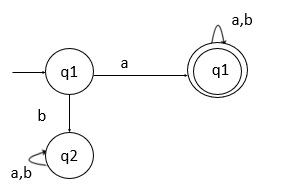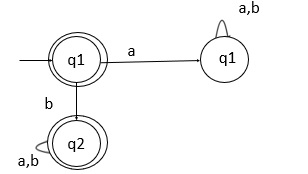# Explain the complementation process in DFA

The complementation process in the deterministic finite automata (DFA) is explained below −

Let’s take a DFA which is defined by (Q, Σ, δ,q0,F) and it accepts the language L1. Now, the DFA that accepts the language L2, where L2 = ̅L1, is defined as follows −

(Q, Σ, δ,q0,Q-F)

The complement of a DFA is obtained by making the non-final states as final states and final states as non-final states.

The language which is accepted by the complemented DFA L2 is the complement of language L1.

## Examples

Let’s consider some examples to get the clarity on the complementation process of DFA.

### Example 1

Consider two languages L1 and L2.

L1={a,ab,aa,aba,aab,aaa,……}

In L2 all strings not starting with ‘a’ over an alphabet {a,b}

L2={€, b,ba,bab,baa,bba,……}

Here we can observe that the two languages are in the form of −

L2 = ̅L1

The transition diagram of DFA for L1 that accepts the set of all strings over {a, b} starting with ‘a’ is given below −q0 on ‘a’ goes to q1 which is a final state and generates the strings that starts with letter ‘a’

{a,ab,aab,abb,aaab,……}

Where q2 is the dead state.

## Construct DFA for the complement

Now, we can construct DFA for the complement simply by interchanging the final and non-final states.

This refers to the change of non-final states as final states and final states as non-final states.

The state transition diagram after constructing DFA for the complement is as follows −The above transition diagram is the complemented DFA which accepts the strings that are not starting with ‘a’.

q0 on ‘a’ goes to q1 which is a dead state so, it is cleared the above state transition DFA will not generate the strings starting with ‘a’.

Updated on: 15-Jun-2021

651 Views